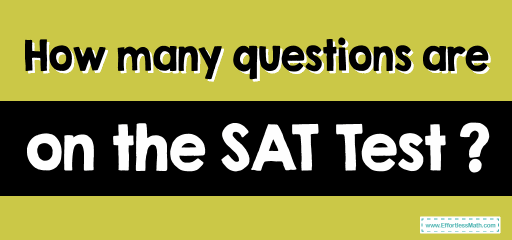# How many questions are on the SAT Test?The SAT is a college entrance exam that many US universities and colleges use as part of their university admissions process. Familiarity with the number of questions in each section of the SAT will help you better prepare for this test. In this article, we answer the question of how many questions are on the SAT. So join us!

## The Absolute Best Book to Ace the SAT Math Test

The SAT includes 154 multiple-choice questions, and the total time is 180 minutes. The SAT is divided into 3 main parts:

• Writing and Language
• Mathematics (two sections)
• Essay (optional)

The Reading section has 52 questions that you have to answer this section in 65 minutes. These 52 questions are related to 5 passages with different lengths. Each section comes with 9-10 questions.

In the Writing section, you answer 44 questions in 35 minutes. Half of these 44 questions are related to grammar and punctuation, and the other half involve general writing strategies, such as writing introductions and conclusions, using appropriate transition words, and analyzing evidence.

The Math section is divided into two sections: no calculator and the calculator section. The section no calculator is first, and you have to answer 20 questions (15 multiple choice and 5 grid-in) in 25 minutes, and the calculator section is in second place and asks 38 questions (30 multiple choice and 8 grid-in) in 55 minutes. In total, you will have 58 questions (45 multiple choice questions and 13 grid-in questions) and 80 minutes for math.

## The Best Books to Ace the SAT Math Test

### What people say about "How many questions are on the SAT Test? - Effortless Math: We Help Students Learn to LOVE Mathematics"?

No one replied yet.

X
30% OFF

Limited time only!

Save Over 30%

SAVE \$5

It was \$16.99 now it is \$11.99